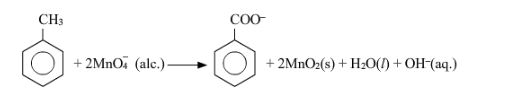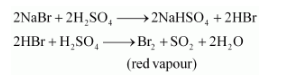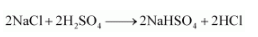# How do you count for the following observations?

Question:

How do you count for the following observations?

(a) Though alkaline potassium permanganate and acidic potassium permanganate both are used as oxidants, yet in the manufacture of benzoic acid from toluene we use alcoholic potassium permanganate as an oxidant. Why? Write a balanced redox equation for the reaction.

(b) When concentrated sulphuric acid is added to an inorganic mixture containing chloride, we get colourless pungent smelling gas HCl, but if the mixture contains bromide then we get red vapour of bromine. Why?

Solution:

(a) In the manufacture of benzoic acid from toluene, alcoholic potassium permanganate is used as an oxidant because of the following reasons.

(i) In a neutral medium, OH ions are produced in the reaction itself. As a result, the cost of adding an acid or a base can be reduced.

(ii) KMnO4 and alcohol are homogeneous to each other since both are polar. Toluene and alcohol are also homogeneous to each other because both are organic compounds. Reactions can proceed at a faster rate in a homogeneous medium than in a heterogeneous medium. Hence, in alcohol, KMnO4 and toluene can react at a faster rate.

The balanced redox equation for the reaction in a neutral medium is give as below:(b) When conc. H2SO4 is added to an inorganic mixture containing bromide, initially HBr is produced. HBr, being a strong reducing agent reduces H2SO4 to SO2 with the evolution of red vapour of bromine.But, when conc. $\mathrm{H}_{2} \mathrm{SO}_{4}$ is added to an inorganic mixture containing chloride, a pungent smelling gas $(\mathrm{HCl})$ is evolved. $\mathrm{HCl}$, being a weak reducing agent, cannot reduce $\mathrm{H}_{2} \mathrm{SO}_{4}$ to $\mathrm{SO}_{2}$.